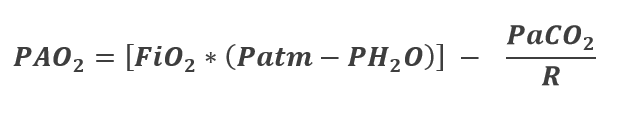4.5.3

# Alveolar Gas Equation

In terms of clinical application, an important equation is the alveolar gas equation. Since it is impractical to stick a probe into the lungs and sample the alveolus to determine the amount of oxygen present, we turn to math. The alveolar gas equation is used to calculate the expected partial pressure of oxygen in the alveolus (PAO2) using the variables expressed in the equation:PAO2 = Partial pressure of oxygen in the alveolus

FiO2 = the fractional concentration of inspired oxygen (expressed as 0.21 for atmospheric air but can be higher if supplemental oxygen is used)

Patm = the atmospheric pressure (sea level = 760 mmHg)

PH2O = the water vapor pressure (usually 47 mmHg at body temperature)

PaCO2 = arterial pressure of carbon dioxide (40 mmHg)

R = the respiratory exchange ratio (0.85)

The respiratory exchange ratio is expressed as:

R = VCO2/VO2

This ratio comes from the interactions of O2 and CO2 and the assumption that inspired air contains no CO2. Although not exactly true, the amount found in normal atmospheric air is so low that it can be considered negligible. This means that any CO2 that is found in the alveolus must have come from metabolism. In reality, the amount of CO2 found is dependent upon the type of fuel the body is burning. For example, if the fuel is carbohydrates than it works out that one molecule of C02 will be produced for one molecule of O2 consumed. This can be seen in the balanced equation below:

However, most western diets are not completely composed of carbohydrates. The R value for most western diets is less than one because the metabolism of fat results in a smaller ratio of CO2 to O2 to metabolize the fat.  This can be seen in the balanced reaction for a common fatty acid called Palmitic acid which is 16 carbons long.

Protein metabolism also yields a ratio smaller than 1 and so when we consider human metabolism of simultaneous carbohydrates, proteins and fats, we find that the true respiratory exchange ratio or “R” averages out very close to .85.

Once we have used the alveolar gas equation to determine the expected alveolar partial pressure of oxygen in the alveolus, we can measure the actual concentration of oxygen in the arterial blood. If the values are remarkably different, we know that there must be a pathology present that is affecting gas exchange.

### End-of-Chapter Survey

: How would you rate the overall quality of this chapter?
1. Very Low Quality
2. Low Quality
3. Moderate Quality
4. High Quality
5. Very High Quality
Comments will be automatically submitted when you navigate away from the page.